# SBI PO 2022: Quantitative Aptitude Quiz-4

## SBI PO 2022: Quantitative Aptitude Quiz- 4

Quantitative Aptitude is a very essential and scoring section of many competitive exams. Candidates appear to be having difficulty with Quantitative Aptitude Questions, so they need to practice more. In this article, we have come up with the SBI PO 2022 Quantitative Aptitude Quiz to enhance your preparation. This SBI PO 2022 Quantitative Aptitude Quiz will assist you to cope with the tough competition. This SBI PO 2022 Quantitative Aptitude Quiz is available to you at no cost. Through this SBI PO 2022 Quantitative Aptitude Quiz, you will learn formulas and short tricks to solve the Quantitative Aptitude Questions. Candidates must solve this SBI PO 2022 Quantitative Aptitude Quiz to improve their exam preparation.

1. Rs. 1750 is given at 14% SI per annum while Rs (1750 + P) is given @ 20% CI per annum. If the difference between both interests at the end of two years is Rs 830. Find P.
(a) 1100
(b) 1150
(c) 1250
(d) 1300
(e) 1350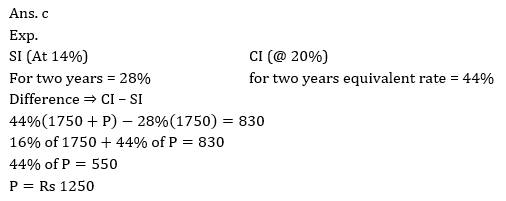2. A and B two articles were sold at 15% profit and 30% profit respectively. If total profit is Rs 84 and if the cost price of A is Rs 40 less than B, find CP of B.
(a) 100
(b) 150
(c) 200
(d) 250
(e) None of these3. Sashi spends 36% of her income in various expenses. Of the remaining, he spends one eigth(1/8)th on her brother while 3/8 th on her sister and remaining keep as savings if the difference betweeen her money spending on brother and sister is Rs 3280. What is Sashi’s saving?
(a) 6480
(b) 6000
(c) 6600
(d) 6560
(e) None of these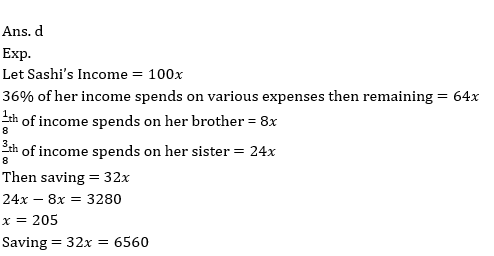4. If 3 is added to the numerator while 1 is subtracted from the denominator, their ratio becomes 5 : 4 while if one is added to the denominator and 5 is subtracted from the numerator the ratio becomes 2 : 3. What is the original fraction?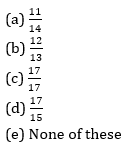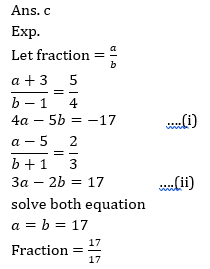5. The batting average for 30 innings of a cricket player is 40 runs. His highest score exceeds his lowest score by 100 runs. If these two innings are not included, the average of the remaining 28 innings is 38 runs. The lowest score of the player is :
(a) 15
(b) 18
(c) 20
(d) 12
(e) None of these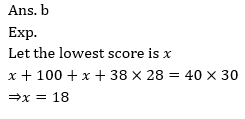Direction (6-10): In each of these questions, two equations (I) and (II) are given. You have to solve both the equations and give answer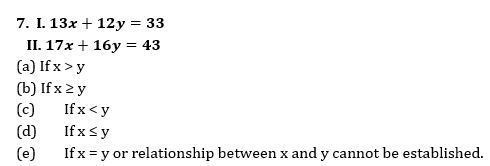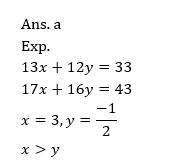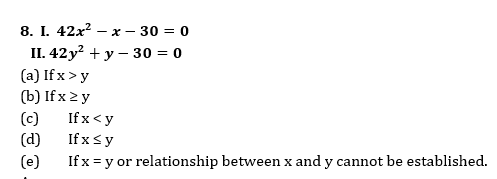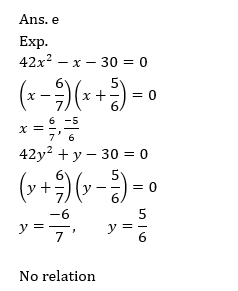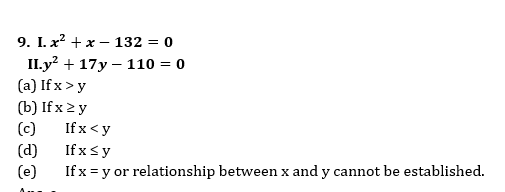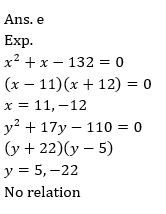#### Attempt Quantitative Aptitude Topic Wise Online Test Series

Recommended PDF’s for:

#### Most important PDF’s for Bank, SSC, Railway and Other Government Exam : Download PDF Now

AATMA-NIRBHAR Series- Static GK/Awareness Practice Ebook PDF Get PDF here
The Banking Awareness 500 MCQs E-book| Bilingual (Hindi + English) Get PDF here
AATMA-NIRBHAR Series- Banking Awareness Practice Ebook PDF Get PDF here
Computer Awareness Capsule 2.O Get PDF here
AATMA-NIRBHAR Series Quantitative Aptitude Topic-Wise PDF Get PDF here
AATMA-NIRBHAR Series Reasoning Topic-Wise PDF Get PDF Here
Memory Based Puzzle E-book | 2016-19 Exams Covered Get PDF here
Caselet Data Interpretation 200 Questions Get PDF here
Puzzle & Seating Arrangement E-Book for BANK PO MAINS (Vol-1) Get PDF here
ARITHMETIC DATA INTERPRETATION 2.O E-book Get PDF here
3